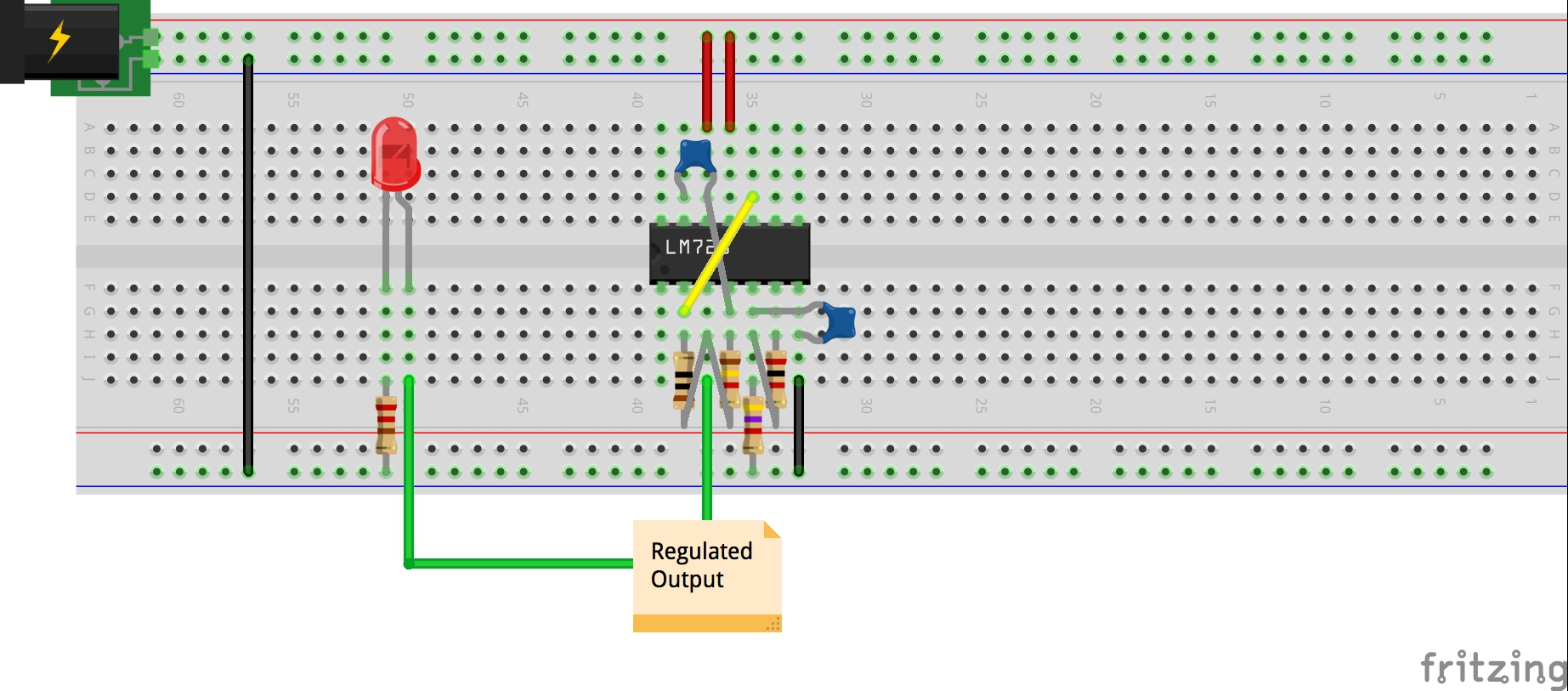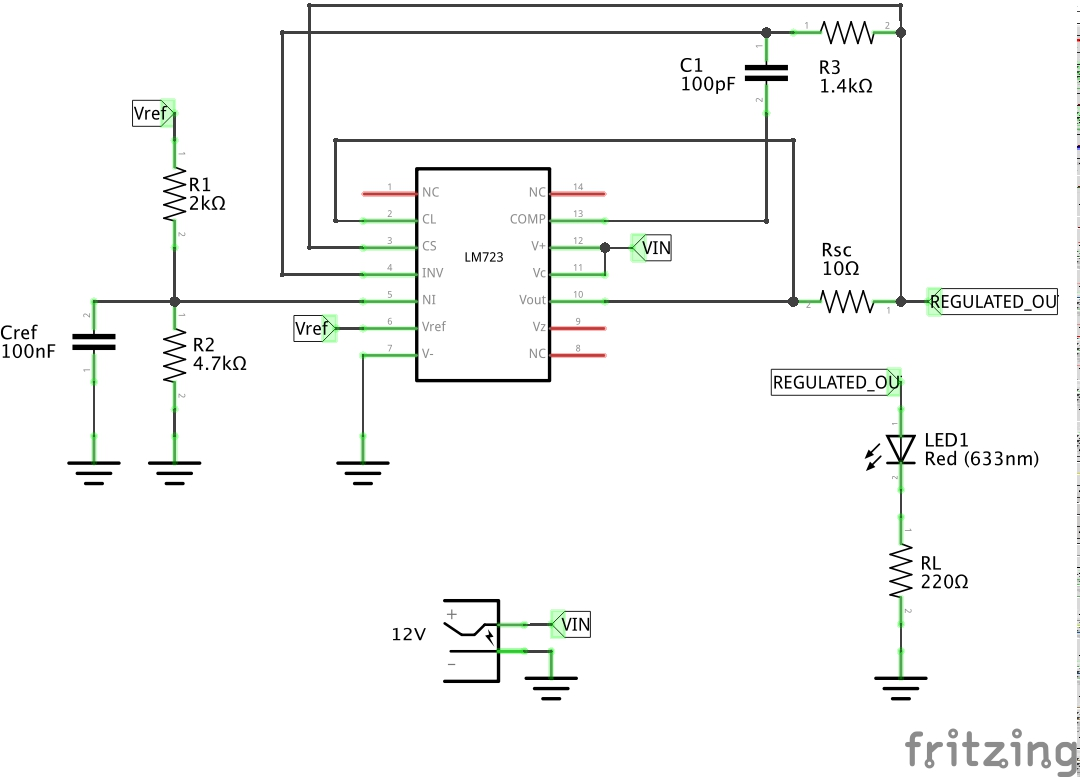# #383 LM723/LowVoltageRegulator

Playing with the basic low voltage output circuit for the LM723 voltage regulator.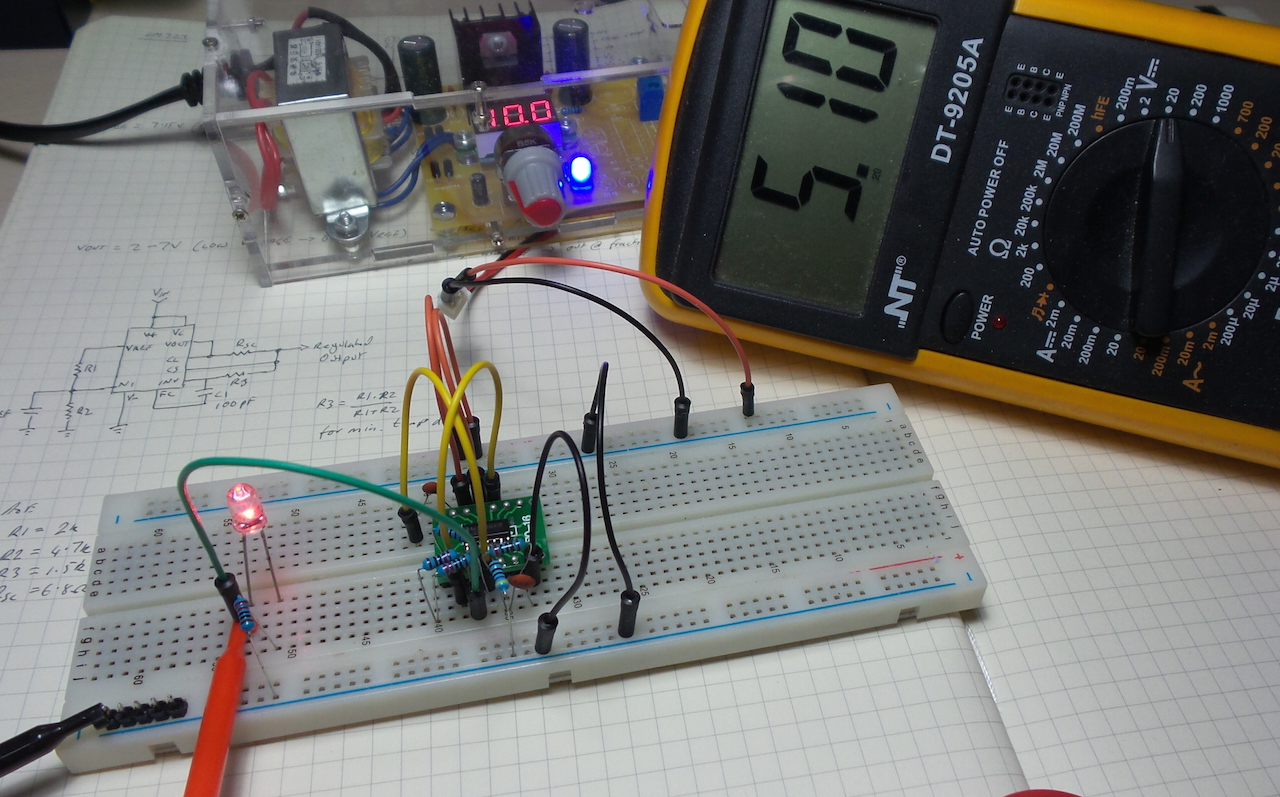The LM723 voltage regulator is a classic IC designed by Bob Widlar. Although not recommended or particularly useful for modern designs, it is widely discussed and documented, so makes an excellent learning tool.

Some great resources for the LM723 are:

This little project is a first dip into the device, with a straight-forward build of the reference circuit for low voltage regulation.

### The LM723 Toolkit

The LM723 is called a toolkit, since it comprises a number of internal modules that must by complements with a (small) number of external components to achieve a range of regulation goals. The basic modules:

• internal voltage reference, 7.15V (typical)
• voltage reference amplifier
• error amplifier
• current limiter
• output control transistor
• output zener diode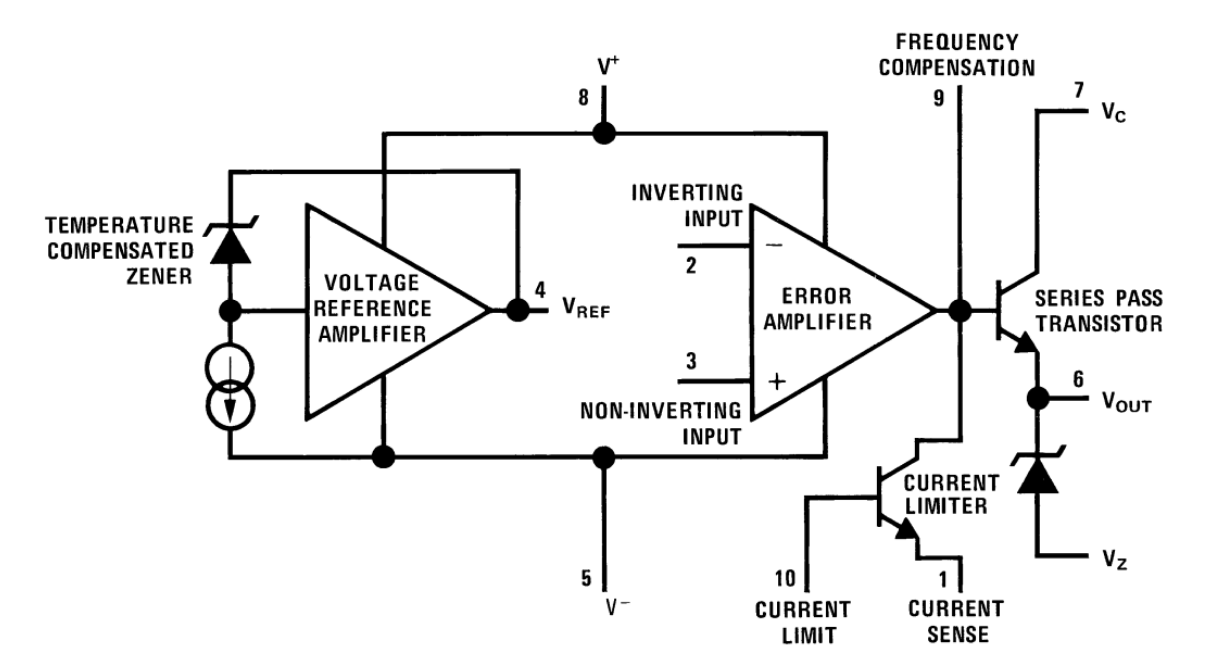### Basic Low Voltage Regulator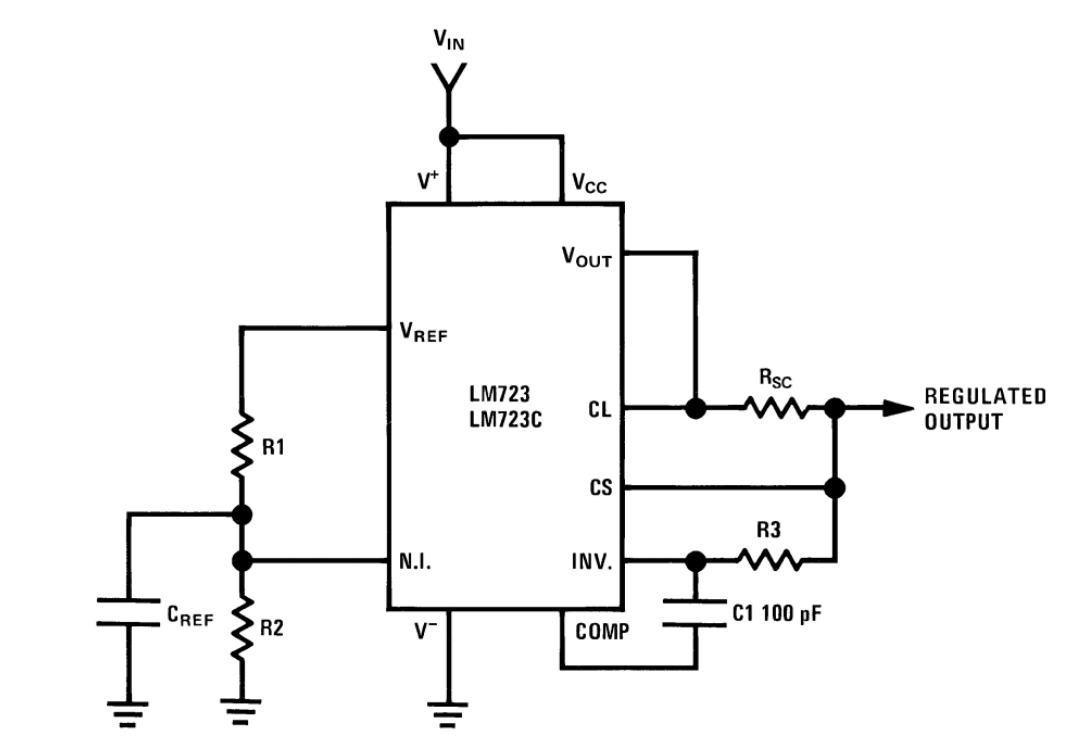#### Output Voltage Set

A resistor divider (R1, R2) is used to set the target voltage as a fraction of the reference voltage. This is what is meant by “low voltage regulator” - the output voltage must be less than the reference voltage. Cref stabilises the reference point.

I’m using R1=2kΩ, and R2=4.7kΩ, so assuming Vref=7.15V, the expected output voltage is Vref * R2/(R1+R2) = 5.016V.

The regulated output is fed back to the error amplifier via R3. For minimum temperature drift, R3 = R1 * R2/(R1+R2) = 1.4kΩ

Actual performance: I’m reading a regulated output of 5.1V, slightly over the predicted value. I measure the actual R1 and R2 values and they are accurate within 10Ω. But I measure the Vref output of the LM723 at 7.27V. At this value, the expected output is indeed 5.10V.

#### Current Limit

Adding current limiting control is optional. It is achieved with a series sense resistor Rsc on the output, used to bridged the current sense and current limit pins, which are effectively the base and emitter pins of a current limiting transistor in the LM723.

When the current through Rsc produces a voltage drop of over about 0.5V, the current limiter starts throttling the output control transistor.

Assuming a limiting drop of 0.5V and a target current limit of 50mA, Rsc = Vbe/Ilimit = 10Ω

I haven’t tested the effectiveness of the current limit yet.

I have an LED and 220Ω current limiting resistor as a dummy load.

### Supply Rail

The 723 requires a relatively high input voltage of between 9.4 and 40 volts (it was designed before “low dropout” was a thing). The main constraint on the minimum voltage is the need to generate the 7.15V reference voltage.

I started running the circuit at 12V, but it works quite happily at 10V (per the pictures). As I reduce the supply voltage, it starts to lose regulation at about 8.9V.

## Construction